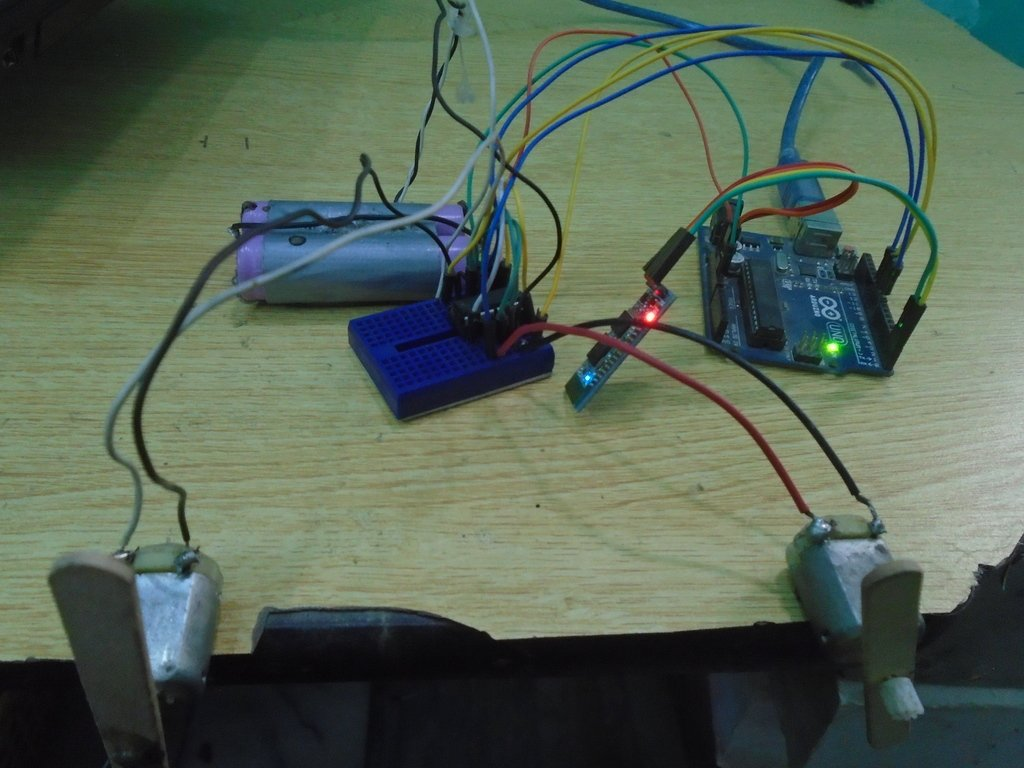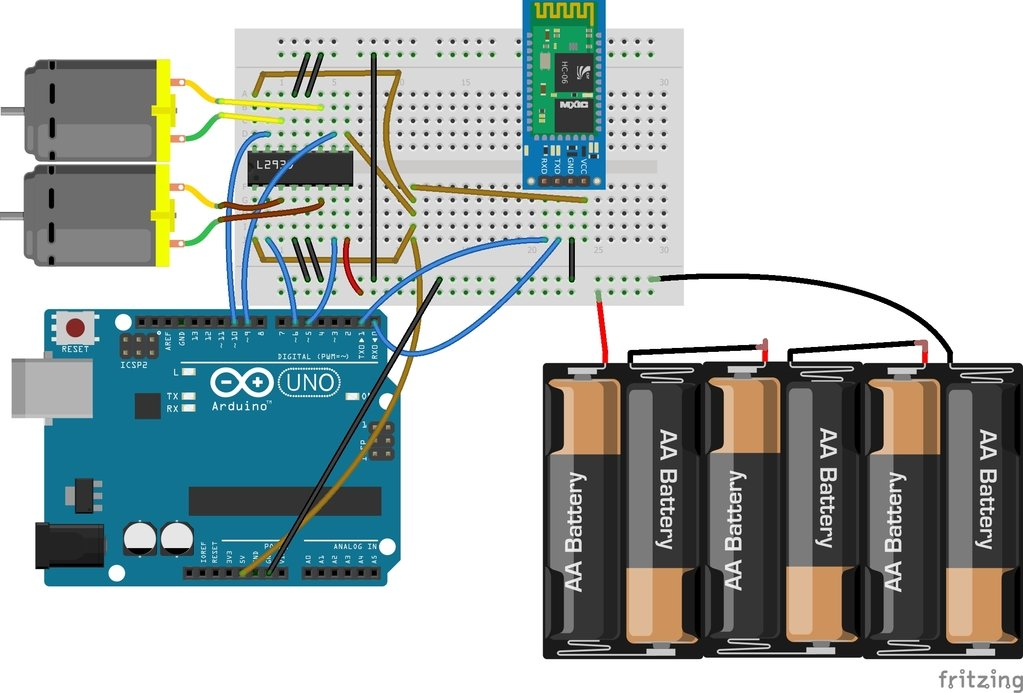# Bluetooth based dc motor speed and direction control using arduino

Bluetooth based dc motor speed and direction control using arduino , In this embedded systems project, we are going to control the motors using the HC-06 Bluetooth device and the android app. Whenever the button will be pressed on the android app, the Arduino will receive the data through the serial communication and will compare this data with the already saved in the data. If the data will match, then the code written inside that function will be executed and the motors will run according to that. The app that we are going to use is the blueterm. I have already posted a project on dc motor speed control with labviewRequired Components for Bluetooth based dc motor speed and direction control

The components required for this project are as follows

you may also like to check : dc motor speed control using pic microcontroller

Circuit Diagram of Bluetooth based dc motor speed and direction controlFirst of all, we will connect the L293D IC with the Arduino. The connections of the L293D motor controller IC with the Arduino are as follows

• Pins 1, 9, 16 of L293D IC with the 5V pin of Arduino.
• Pin 2 of L293D IC is the Input pin; connect it with the pin 6 of Arduino.
• Pin 3 of L293D IC to one end of the motor and connect the other end of the motor to the pin 6 of L293D.
• Pins 4, 5, 12 and 13 are GND pins of L293D IC; connect these to the GND of Arduino.
• Pin 7 of L293D IC is the Input pin; connect it with the pin 5 of Arduino.
• Pin 8 is the VCC pin of L293D IC, connect it to positive end of battery and connect the negative end of battery to the Ground.
• Pin 10 of L293D IC is the Input pin for second motor; connect it with pin 9 of Arduino.
• Pin 11 of L293D to one end of motor and connect the second end of motor to the pin 14 of L293D.
• Pin 15 of L293D is the second Input pin for second motor; connect it with the pin 10 of Arduino.

After that, make the connections for the Bluetooth device HC-06 with Arduino. The connections of the Bluetooth device HC-06 with Arduino are as follows

• VCC of HC-06 to the 5V of Arduino
• GND of HC-06 to the GND of Arduino
• TX of HC-06 to the RX pin of Arduino
• RX pin of HC-06 to the TX pin of Arduino

Working of Bluetooth based dc motor speed and direction control

The HC-06 Bluetooth module works with the Arduino through the serial communication which means that the Arduino will send and receive the data through the Serial.To send the information from the App, we first need to install it. You can install the App from here. After installing the APP, open it and from the options and connect it to the Bluetooth module. After connecting it to the Bluetooth module, it will show you blue empty screen. Now, when you will type ‘1’ there, then the left motor will start to move and when you will type ‘2’, then the right motor will start to move and similarly by typing ‘3’, the both the motors will turn clockwise and by typing ‘4’ , both the motors will turn anticlockwise. By typing ‘0’, both the motors will stop to move.

if you don’t know how to interface how to interface HC 06 BT module with Arduino check this: HC-05 Bluetooth module interfacing with Arduino

Code of Bluetooth based dc motor speed and direction control

```int first_motor_pin1 = 11;

int first_motor_pin2 = 10;

int second_motor_pin1 = 9;

int second_motor_pin2 = 8;

int state;

int flag = 0;

void setup ( ) {

Serial.begin (9600);

pinMode (first_motor_pin1, OUTPUT);

pinMode (first_motor_pin2, OUTPUT);

pinMode (second_motor_pin1, OUTPUT);

pinMode (second_motor_pin2, OUTPUT);

}

void loop ( ) {

if(Serial.available( ) > 0){

flag = 0;

}

if (state == '1') {

digitalWrite (first_motor_pin1, LOW);

digitalWrite (first_motor_pin2, HIGH);

digitalWrite (second_motor_pin1, HIGH);

digitalWrite (second_motor_pin2, HIGH);

if(flag == 0){

Serial.println("Left Motor ON");

flag = 1;

}

}

else if (state == '2') {

digitalWrite (first_motor_pin1, HIGH);

digitalWrite (first_motor_pin2, HIGH);

digitalWrite (second_motor_pin1, HIGH);

digitalWrite (second_motor_pin2, LOW);

if(flag == 0){

Serial.println("Right Motor ON");

flag = 1;

}

}

else if (state == '3') {

digitalWrite (first_motor_pin1, LOW);

digitalWrite (first_motor_pin2, HIGH);

digitalWrite (second_motor_pin1, HIGH);

digitalWrite (second_motor_pin2, LOW);

if(flag == 0){

Serial.println("Both Motors Clockwise");

flag = 1;

}

}

else if (state == '4') {

digitalWrite (first_motor_pin1, HIGH);

digitalWrite (first_motor_pin2, LOW);

digitalWrite (second_motor_pin1, LOW);

digitalWrite (second_motor_pin2, HIGH);

if(flag == 0){

Serial.println("Both Motors Anti-clockwise");

flag = 1;

}

}

else if (state == '0') {

digitalWrite (first_motor_pin1, LOW);

digitalWrite (first_motor_pin2, LOW);

digitalWrite (second_motor_pin1, LOW);

digitalWrite (second_motor_pin2, LOW);

if(flag == 0){

Serial.println("Both Motors OFF");

flag = 1;

}

}

}```

Code Explanation

First of all, we initialized the pins in the code which are for controlling the motors. We initialized four pins, two are for controlling the first motor and the other two are for controlling the second motor. The state variable we initialized is for storing the output that will come from the Bluetooth device. The flag variable initialized will make sure that the serial prints only once the state.

```int first_motor_pin1 = 11;

int first_motor_pin2 = 10;

int second_motor_pin1 = 9;

int second_motor_pin2 = 8;

int state;

int flag = 0;```

Then in the setup function, we declared all the pins as the output pins because we will give the output to the L293D motor controller from these pins. This output from the Arduino will be the input for the L293D motor controller IC.

``` pinMode (first_motor_pin1, OUTPUT);

pinMode (first_motor_pin2, OUTPUT);

pinMode (second_motor_pin1, OUTPUT);

pinMode (second_motor_pin2, OUTPUT);```

Then in the loop function, we checked that if the data is available or not or if the button on the android app is pressed or not. If the data will be available, then it will get stored in the state variable. Then we will compare this data with the already saved data in our code. If the data will match, then the code written in that will get execute and the motor will run according to that.

```if(Serial.available( ) > 0){

flag = 0;

}

if (state == '1') {

digitalWrite (first_motor_pin1, LOW);

digitalWrite (first_motor_pin2, HIGH);

digitalWrite (second_motor_pin1, HIGH);

digitalWrite (second_motor_pin2, HIGH);

if(flag == 0){

Serial.println("Left Motor ON");

flag = 1;

}

}```

### 1 thought on “Bluetooth based dc motor speed and direction control using arduino”

1. Where is speed control.